Courses

# Facts that Matter- Coordinate Geometry Class 10 Notes | EduRev

## Class 10 : Facts that Matter- Coordinate Geometry Class 10 Notes | EduRev

The document Facts that Matter- Coordinate Geometry Class 10 Notes | EduRev is a part of the Class 10 Course Class 10 Mathematics by VP Classes.
All you need of Class 10 at this link: Class 10

Facts that Matter

• Distance Formula
I. The distance between two points P (x1, y1) and Q (x2, y2) is given by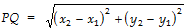II. The distance of P (x, y) from the origin is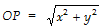• Section Formula
I. If P (x, y) divides the line segment AB, joining the points A (x1, y1) and B (x2, y2) in the ratio m : n, then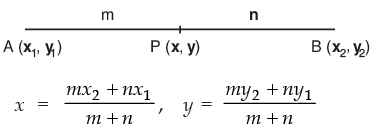II. The coordinates (x, y) of the mid-point of PQ are given by: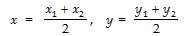III. If A (x1, y1), B (x2, y2) and C (x3, y3) be the vertices of ΔABC, then the coordinates G (x, y) of the centroid of ΔABC are: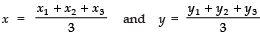Offer running on EduRev: Apply code STAYHOME200 to get INR 200 off on our premium plan EduRev Infinity!

132 docs

,

,

,

,

,

,

,

,

,

,

,

,

,

,

,

,

,

,

,

,

,

;